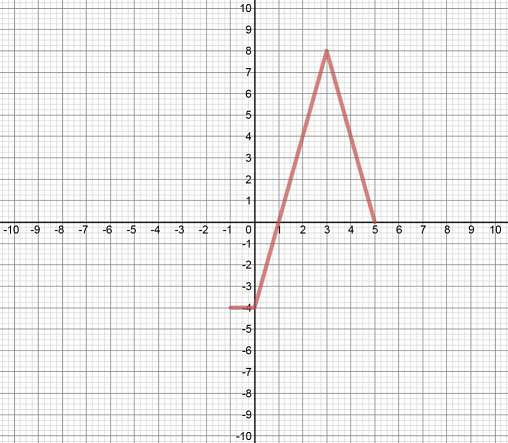# Combining transformations of functions

### Combining transformations of functions

#### Lessons

• 1.
Describe the Combination of Transformations
Compared to $y = f\left( x \right)$, describe every step of transformations applied to:
$y = - 2f\left[ {3\left( {x + 4} \right)} \right] + 5$

• 2.
Write the Equation of a Transformed Function
Transform the function $f\left( x \right) = \frac{1}{x}$ into the function $g\left( x \right)$ by:
a)
stretching horizontally by a factor of 2 about the y-axis

b)
stretching vertically by a factor of $\frac{3}{5}$ about the x-axis

c)
vertical translation of 7 units up

d)
reflection in the y-axis

e)
horizontal translation of 4 units to the left

f)
reflection in the x-axis

Write the function for $g(x)$.

• 3.
Use "Coordinate Mapping Formula" to Graph a Transformed Function
Given the graph of $y = f\left( x \right)$ as shown,
a)
describe every step of transformations applied to: $y = \frac{1}{4}f\left( {3 - \frac{x}{2}} \right) - 1$

b)
Graph the transformed function on the same set of coordinate axes.

c)
Shortcut: use "Coordinate Mapping Formula" to graph the transformed function.